# CBSE Class 10 Mathematics (Standard) Sample Paper 2022-23# CBSE Sample Question Papers Class 10 Mathematics Standard Book For 2023 Board Exam

CBSE Class 10 Mathematics (Standard) Sample Paper 2022-23: Download CBSE 10 Mathematics (Standard) Sample Question Paper PDF Here CBSE Class 10 Maths Sample Paper 2022-23 CBSE has released Class 10 Mathematics  (Standard) Sample Question Paper. Download direct CBSE Class 10 Mathematics  (Standard) Sample Question Paper pdf here. Visit official website at  cbse.gov.in or cbseacademic.in.

general instructions provided in the CBSE Class 10 Mathematics  (Standard) Sample Question Paper 2022-23 listed below:

### CBSE Class 10 Mathematics (Standard) Sample Paper Question 2022-23

General Instructions:

1. This Question Paper has 5 Sections A-E.
2. Section A has 20 MCQs carrying 1 mark each
3. Section B has 5 questions carrying 02 marks each.
4. Section C has 6 questions carrying 03 marks each.
5. Section D has 4 questions carrying 05 marks each.
6. Section E has 3 case based integrated units of assessment (04 marks each) with subparts of the values of 1, 1 and 2 marks each respectively.
7. All Questions are compulsory. However, an internal choice in 2 Qs of 5 marks, 2 Qs of 3 marks and 2 Questions of 2 marks has been provided. An internal choice has been provided in the 2marks questions of Section E
8. Draw neat figures wherever required. Take π =22/7 wherever required if not stated.

Candidates appearing for  CBSE Class 10 Mathematics  (Standard) board exam 2022-23 can find below the CBSE Class 10 Mathematics  (Standard) Sample Question Paper 2022-23 to prepare for the CBSE Class 10 Mathematics  (Standard) Board Exam 2022-23.

### CBSE Sample Paper Class 10 Maths Standard 2022-2023

SECTION – A

1. Let a and b be two positive integers such that a = p3q4 and b = p2q3 , where p and q are prime numbers. If HCF(a, b) = pmqn and LCM(a, b) = prqs , then (m + n)(r + s) =

(a) 15

(b) 30

(c) 35

(d) 72

2. Let p be a prime number. The quadratic equation having its roots as factors of p is

(a) x2 –px +p=0

(b) x2 –(p+1)x +p=0

(c) x2+(p+1)x +p=0

(d) x2 –px+p+1=0

3. If α and β are the zeros of a polynomial f(x) = px2 – 2x + 3p and α + β = αβ, then p is

(a) -2/3

(b) 2/3

(c) 1/3

(d) -1/3

4. If the system of equations 3x+y =1 and (2k-1)x +(k-1)y =2k+1 is inconsistent, then k =

(a) -1

(b) 0

(c) 1

(d) 2

5. If the vertices of a parallelogram PQRS taken in order are P(3,4), Q(-2,3) and R(-3,-2), then the coordinates of its fourth vertex S are

(a) (-2,-1)

(b) (-2,-3)

(c) (2,-1)

(d) (1,2)

6. ∆ABC~∆PQR. If AM and PN are altitudes of ∆ABC and ∆PQR respectively and AB2 : PQ2 = 4 : 9, then AM: PN =

(a) 3:2

(b) 16:81

(c) 4:9

(d) 2:3

7. If x tan 60° cos 60°= sin60° cot 60° , then x =

(a) cos30°

(b) tan30°

(c) sin30°

(d) cot30°

8. If sinθ + cosθ = √2, then tanθ + cot θ =

(a) 1

(b) 2

(c) 3

(d) 4

9. In the given figure, DE ∥ BC, AE = a units, EC =b units, DE =x units and BC = y units. Which of the following is true?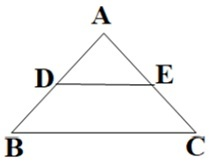(a) x= 𝑎+𝑏/𝑎𝑦

(b) y= 𝑎𝑥/𝑎+𝑏

(c) x= 𝑎𝑦/𝑎+𝑏

(d) 𝑥/𝑦 = 𝑎/b

10. ABCD is a trapezium with AD ∥ BC and AD = 4cm. If the diagonals AC and BD intersect each other at O such that AO/OC = DO/OB =1/2, then BC =

(a) 6cm

(b) 7cm

(c) 8cm

(d) 9cm

11. If two tangents inclined at an angle of 60ᵒ are drawn to a circle of radius 3cm, then the length of each tangent is equal to

(a) 3√3/2 cm

(b) 3cm

(c) 6cm

(d) 3√3cm

12. The area of the circle that can be inscribed in a square of 6cm is

(a) 36π cm2

(b) 18π cm2

(c) 12 π cm2

(d) 9π cm2

13. The sum of the length, breadth and height of a cuboid is 6√3cm and the length of its diagonal is 2√3cm. The total surface area of the cuboid is

(a) 48 cm2

(b) 72 cm2

(c) 96 cm2

(d) 108 cm2

14. If the difference of Mode and Median of a data is 24, then the difference of median and mean is

(a) 8

(b) 12

(c) 24

(d) 36

15. The number of revolutions made by a circular wheel of radius 0.25m in rolling a distance of 11km is

(a) 2800

(b) 4000

(c) 5500

(d) 7000

16. For the following distribution,

 Class 0-5 5-10 10-15 15-20 20-25 Frequency 10 15 12 20 9

the sum of the lower limits of the median and modal class is

(a) 15

(b) 25

(c) 30

(d) 35

17. Two dice are rolled simultaneously. What is the probability that 6 will come up at least once?

(a)1/6

(b) 7/36

(c) 11/36

(d) 13/36

18. If 5 tanβ =4, then 5 𝑠𝑖𝑛𝛽−2 cos𝛽/5 sin𝛽+2 cos𝛽 =

(a) 1/3

(b) 2/5

(c) 3/5

(d) 6

DIRECTION: In the question number 19 and 20, a statement of assertion (A) is followed by a statement of Reason (R). Choose the correct option

19. Statement A (Assertion): If product of two numbers is 5780 and their HCF is 17, then their LCM is 340

Statement R( Reason) : HCF is always a factor of LCM

(a) Both assertion (A) and reason (R) are true and reason (R) is the correct explanation of assertion (A)

(b) Both assertion (A) and reason (R) are true and reason (R) is not the correct explanation of assertion (A)

(c) Assertion (A) is true but reason (R) is false.

(d) Assertion (A) is false but reason (R) is true.

20. Statement A (Assertion): If the co-ordinates of the mid-points of the sides AB and AC of ∆ABC are D(3,5) and E(-3,-3) respectively, then BC = 20 units

Statement R( Reason): The line joining the mid points of two sides of a triangle is parallel to the third side and equal to half of it.

(a) Both assertion (A) and reason (R) are true and reason (R) is the correct explanation of assertion (A)

(b) Both assertion (A) and reason (R) are true and reason (R) is not the correct explanation of assertion (A)

(c) Assertion (A) is true but reason(R) is false.

(d) Assertion (A) is false but reason(R) is true.

SECTION – B

21. If 49x+51y= 499, 51 x+49 y= 501, then find the value of x and y

22. In the given figure below, AD/AE = AC/BD and ∠1 = ∠2. Show that ∆ BAE~ ∆CAD .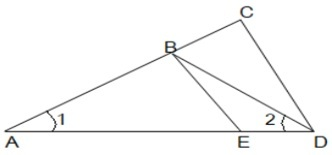23. In the given figure, O is the centre of circle. Find ∠AQB, given that PA and PB are tangents to the circle and ∠APB= 75°.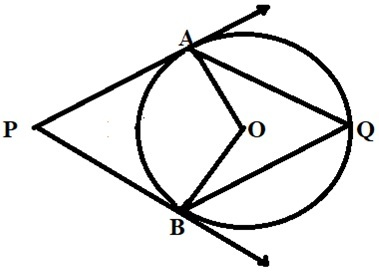24. The length of the minute hand of a clock is 6cm. Find the area swept by it when it moves from 7:05 p.m. to 7:40 p.m.

OR

In the given figure, arcs have been drawn of radius 7cm each with vertices A, B, C and D of quadrilateral ABCD as centres. Find the area of the shaded region.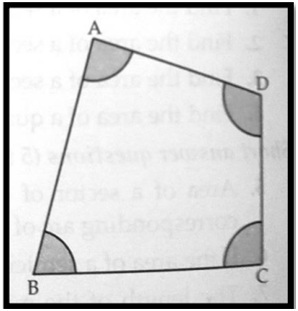25. If sin(A+B) =1 and cos(A-B)= √3/2, 0°< A+B ≤ 90° and A> B, then find the measures of angles A and B.

OR

Find an acute angle θ when cosθ − sin θ/cosθ + sin θ = 1 − √3/1 + √3

SECTION – C

26. Given that √3 is irrational, prove that 5 + 2√3 is irrational.

27. If the zeroes of the polynomial x2 + px +q are double in value to the zeroes of the polynomial 2x2 – 5x – 3, then find the values of p and q.

28. A train covered a certain distance at a uniform speed. If the train would have been 6 km/h faster, it would have taken 4 hours lessthan the scheduled time. And, if the train were slower by 6 km/hr ; it would have taken 6 hours more than the scheduled time. Find the length of the journey.

OR

Anuj had some chocolates, and he divided them into two lots A and B. He sold the first lot at the rate of Rs. 2 for 3 chocolates and the second lot at the rate of Rs. 1 per chocolate, and got a total of Rs. 400. If he had sold the first lot at the rate of Rs. 1 per chocolate, and the second lot at the rate of Rs. 4 for 5 chocolates, his total collection would have been Rs. 460. Find the total number of chocolates he had.

29. Prove the following that-

tan3θ/1+ tan2θ + cot3θ/1 + cot2θ = secθ cosecθ – 2 sinθ cosθ

30. Prove that a parallelogram circumscribing a circle is a rhombus

OR

In the figure XY and X’Y’ are two parallel tangents to a circle with centre O and another tangent AB with point of contact C interesting XY at A and X’Y’ at B, what is the measure of ∠AOB.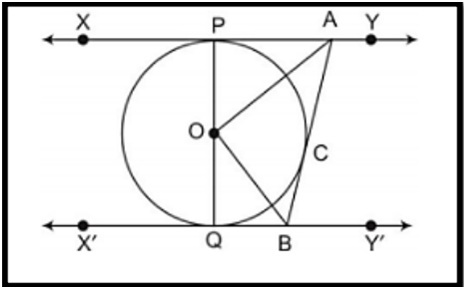31. Two coins are tossed simultaneously. What is the probability of getting

(ii) At most one tail?

(iii) A head and a tail?

SECTION – D

32. To fill a swimming pool two pipes are used. If the pipe of larger diameter used for 4 hours and the pipe of smaller diameter for 9 hours, only half of the pool can be filled. Find, how long it would take for each pipe to fill the pool separately, if the pipe of smaller diameter takes 10 hours more than the pipe of larger diameter to fill the pool?

OR

In a flight of 600km, an aircraft was slowed down due to bad weather. Its average speed for the trip was reduced by 200 km/hr from its usual speed and the time of the flight increased by 30 min. Find the scheduled duration of the flight.

33. Prove that if a line is drawn parallel to one side of a triangle intersecting the other two sides in distinct points, then the other two sides are divided in the same ratio.

Using the above theorem prove that a line through the point of intersection of the diagonals and parallel to the base of the trapezium divides the non parallel sides in the same ratio.

34. Due to heavy floods in a state, thousands were rendered homeless. 50 schools collectively decided to provide place and the canvas for 1500 tents and share the whole expenditure equally. The lower part of each tent is cylindrical with base radius 2.8 m and height 3.5 m and the upper part is conical with the same base radius, but of height 2.1 m. If the canvas used to make the tents costs Rs. 120 per m2 , find the amount shared by each school to set up the tents.

OR

There are two identical solid cubical boxes of side 7cm. From the top face of the first cube a hemisphere of diameter equal to the side of the cube is scooped out. This hemisphere is inverted and placed on the top of the second cube’s surface to form a dome. Find

(i) the ratio of the total surface area of the two new solids formed

(ii) volume of each new solid formed.

35. The median of the following data is 525. Find the values of x and y, if the total frequency is 100

 Class interval Frequency 0−100 2 100−200 5 200−300 x 300−400 12 400−500 17 500−600 20 600−700 y 700−800 9 800−900 7 900−1000 4

SECTION – E

Case study based questions are compulsory.

36. A tiling or tessellation of a flat surface is the covering of a plane using one or more geometric shapes, called tiles, with no overlaps and no gaps. Historically, tessellations were used in ancient Rome and in Islamic art. You may find tessellation patterns on floors, walls, paintings etc. Shown below is a tiled floor in the archaeological Museum of Seville, made using squares, triangles and hexagons.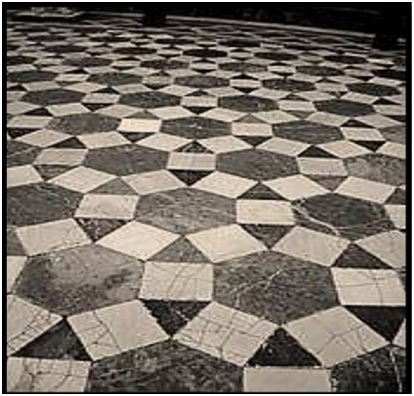A craftsman thought of making a floor pattern after being inspired by the above design. To ensure accuracy in his work, he made the pattern on the Cartesian plane. He used regular octagons, squares and triangles for his floor tessellation pattern.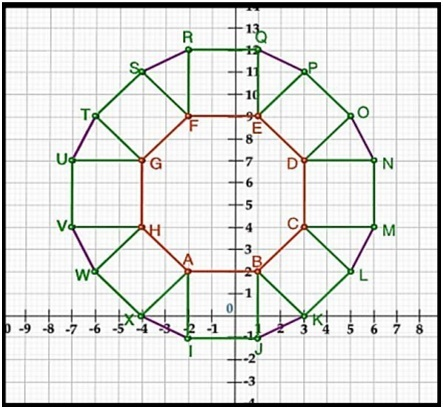Use the above figure to answer the questions that follow:

(i) What is the length of the line segment joining points B and F?

(ii) The centre ‘Z’of the figure will be the point of intersection of the diagonals of quadrilateral WXOP. Then what are the coordinates of Z?

(iii) What are the coordinates of the point on y axis equidistant from A and G?

OR

What is the area of Trapezium AFGH?

37. The school auditorium was to be constructed to accommodate at least 1500 people. The chairs are to be placed in concentric circular arrangement in such a way that each succeeding circular row has 10 seats more than the previous one.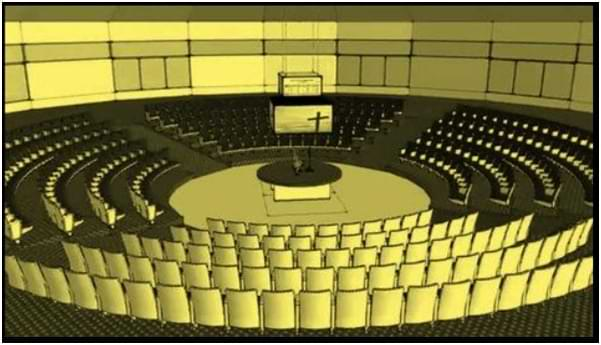(i) If the first circular row has 30 seats, how many seats will be there in the 10th row?

(ii) For 1500 seats in the auditorium, how many rows need to be there?

OR

If 1500 seats are to be arranged in the auditorium, how many seats are still left to be put after 10th row?

(iii) If there were 17 rows in the auditorium, how many seats will be there in the middle row?

38. We all have seen the airplanes flying in the sky but might have not thought of how they actually reach the correct destination. Air Traffic Control (ATC) is a service provided by ground-based air traffic controllers who direct aircraft on the ground and through a given section of controlled airspace, and can provide advisory services to aircraft in non-controlled airspace. Actually, all this air traffic is managed and regulated by using various concepts based on coordinate geometry and trigonometry.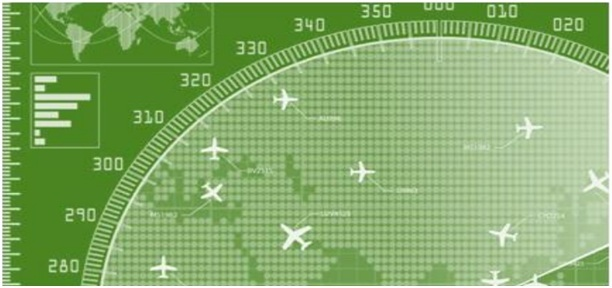At a given instance, ATC finds that the angle of elevation of an airplane from a point on the ground is 60°. After a flight of 30 seconds, it is observed that the angle of elevation changes to 30°. The height of the plane remains constantly as 3000√3 m. Use the above information to answer the questions that follow-

(i) Draw a neat labelled figure to show the above situation diagrammatically.

(ii) What is the distance travelled by the plane in 30 seconds?

OR

Keeping the height constant, during the above flight, it was observed that after 15(√3 -1) seconds, the angle of elevation changed to 45°. How much is the distance travelled in that duration.

(iii) What is the speed of the plane in km/hr.

CBSE Class 10 Mathematics (Standard) Sample Paper 2022-23 in pdf format for you to download and print it according to your convenience.